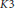Hostname: page-component-7d684dbfc8-dh8xm Total loading time: 0 Render date: 2023-09-29T10:15:31.078Z Has data issue: false Feature Flags: { "corePageComponentGetUserInfoFromSharedSession": true, "coreDisableEcommerce": false, "coreDisableSocialShare": false, "coreDisableEcommerceForArticlePurchase": false, "coreDisableEcommerceForBookPurchase": false, "coreDisableEcommerceForElementPurchase": false, "coreUseNewShare": true, "useRatesEcommerce": true } hasContentIssue false

# A Mahler Measure of a K3 Surface Expressed as a Dirichlet L-Series

Published online by Cambridge University Press:  20 November 2018

## Abstract

Core share and HTML view are not possible as this article does not have html content. However, as you have access to this content, a full PDF is available via the ‘Save PDF’ action button.

We present another example of a 3-variable polynomial defining a$K3$-hypersurface and having a logarithmic Mahler measure expressed in terms of a Dirichlet$L$-series.

Type
Research Article
Information
Canadian Mathematical Bulletin , 01 March 2012 , pp. 26 - 37

## References

 Beilinson, A., Higher regulators of modular curves. In: Application of Algebraic K-theory to Algebraic Geometry and Number Theory. Contemp. Math. 55, American Mathematical Society, Providence, RI, 1986, pp. 134.Google Scholar
 Bertin, M. J., Une mesure de Mahler explicite. C. R. Acad. Sci. Paris Sér. I Math. 333(2001), no. 1, 13.CrossRefGoogle Scholar
 Bertin, M. J., Mesure de Mahler d’hypersurfaces K3 . J. Number Theory 128(2008), no. 11, 28902913. doi:10.1016/j.jnt.2007.12.012CrossRefGoogle Scholar
 Bertin, M. J., Mahler's measure and L-series of K3 hypersurfaces. In: Mirror Symmetry V. AMS/IP Studies in Advanced Mathematics 38, American Mathematical Society, Providence, RI, 2006, pp. 318.Google Scholar
 Bertin, M. J., The Mahler measure and the L-series of a singular K3-surface. arXiv:0803.0413v1math.NT(math.AG).Google Scholar
 Boyd, D. W., Mahler's measure and special values of L-functions. Experiment. Math. 7(1998), no. 1, 3782.CrossRefGoogle Scholar
 Elkies, N. and Schütt, M., Modular forms and K3 surfaces. arXiv:math.AG/0809.0830v1.Google Scholar
 Hulek, K., Kloosterman, R., and Schütt, M., Modularity of Calabi-Yau varieties. In: Global Aspects of Complex Geometry. Springer, Berlin, 2006, pp. 271309.CrossRefGoogle Scholar
 Lalin, M. and Rogers, M., Functional equations for Mahler measures of genus-one curves. Algebra Number Theory 1(2007), no. 1, 87117. doi:10.2140/ant.2007.1.87CrossRefGoogle Scholar
 Livné, R., Cubic exponential sums and Galois representations. In: Current Trends in Arithmetical Algebraic Geometry. Contemp. Math. 67, American Mathematical Society, Providence, RI, 1987, pp. 247261.CrossRefGoogle Scholar
 Livné, R., Motivic orthogonal two-dimensional representations of. Israel J. of Math 92(1995), no. 1-3, 149156. doi:10.1007/BF02762074CrossRefGoogle Scholar
 Peters, C., Top, J., and van der Vlugt, M., The Hasse zeta function of a K3 surface related to the number of words of weight5 in the Melas codes. J. Reine Angew. Math. 432(1992), 151176.Google Scholar
 Peters, C., Top, J., and van der Vlugt, M., Modular Mahler measures. I. In: Topics in Number Theory. Math. Appl. 467, Kluwer, Dordrecht, 1999, pp. 1748.Google Scholar
 Shioda, T., On elliptic modular surfaces. J. Math. Soc. Japan 24(1972), 2059. doi:10.2969/jmsj/02410020CrossRefGoogle Scholar
 Schütt, M., CM newforms with rational coefficients. arXiv:math.NT/0511228v5.Google Scholar
 Williams, K., Some Lambert series expansions of products of theta functions. Ramanujan Journal 3(1999), no. 4, 367384. doi:10.1023/A:1009853106329CrossRefGoogle Scholar
 Yui, N., Arithmetic of certain Calabi-Yau varieties and mirror symmetry. In: Arithmetic Algebraic Geometry. IAS/Park City Math. Ser. 9, American Mathematical Society, Providence, RI, 2001, pp. 507569.Google Scholar
 Zagier, D. and Gangl, H., Classical and elliptic polylogarithms and special values of L-series. In: The Arithmetic and Geometry of Algebraic Cycles. Nato Sciences Series C 548, NATO Sci. Ser. C Math. Phys. Sci. 548, Kluwer, Dordrecht, 2000, pp. 561615.CrossRefGoogle Scholar
 Zucker, I. and McPhedran, R., Dirichlet L-series with real and complex characters and their application to solving double sums. arXiv:0708.1224v1[math-ph]. doi:10.1098/rspa.2007.0162CrossRefGoogle Scholar
 Zucker, I. and Robertson, M., A systematic approach to the evaluation of Σ (m,n≠0,0)(am 2 + bmn + cn 2 )s . J. Phys. A: 9(1976), no. 8, 12151225. doi:10.1088/0305-4470/9/8/007CrossRefGoogle Scholar